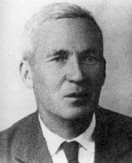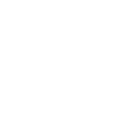What is random?

Below are three different pictures, each containing a few hundred dots. In which pictures would you consider the dots to arranged randomly?

Clearly the image on the left is not random, the points are distributed on a grid.

In the center image, the points are not in a structured grid, but they’re also not random; they’ve been arranged so that they are fairly uniformly distributed and the density of the points is pretty consistent. To do this, placement of a point is dependant on others. Many people, because of superstition, or belief in some bizarre “conservation of luck”, or just thinking that random means “spreading out”, are not rational in their understanding of randomness.

Some people seem to get uncomfortable with clusters, somehow thinking that clusters are ‘less random’. When selecting lottery ticket numbers these people will not group numbers together, thinking that runs of numbers are less likely. Others think that if a coin lands many times on heads it is ‘more likely’ to land tails in the future to help balance out the luck (as if the coin had any kind of memory of past flips!)

Out of the three, the image on the right is the ‘random’ one. If you look closely, you can see clusters of points and gaps/voids. This is what we’d expect from random. As each point is placed, it is added independently of any of the other existing points (truly randomly). In the middle image, there are no clusters or voids as points were added based on proximity to others to stop these occurring.

## Random

Let’s simplify things and look at strings of digits instead of 2D coordinates. As we’ll see, a way to decide if something is random is how we could describe it. We will look at series of strings of 1’s and 0’s to determine if we think each is ‘random’ or not.

First an easy one, is the string below random?

11111111111111110000000000000000

No, we can see there is a pattern to it. Put in another way, we can describe this string in a more compact (efficient) way because it has structure. We can compress it. The pseudo code below is a an alternative way of describing the string above.

‘1’16+’0’16

If we look at this ‘regular expression like’ piece of code above we can see that it describes the longer string perfectly. The length of this short describing program (even with redundant quotes!) is shorter than the output. We’ve been able to compress the original string as it had structure and repetition.

Let’s look at another example. For the string below …

10101010101010101010101010101010

… we can represent as the shorter:

‘10’*16

Similarly …

11010101010101011101010101010101

… can be represented by the shorter pseudo program:

‘1101010101010101’*2

or even:

(‘11’+’01’7)2

However, the following string is a bit of a mess.

11010100110110101010011111010101

It doesn’t really have any structure and, if we had to write a program to describe this string, the program itself would be longer than the string so the most efficient way of describing this string is to simply quote the string itself. This gives us a good definition of randomness. Something that is truly random has no structure and thus is not describable by any formula better than itself. It is incompressible.

This does not mean that a string of random digits cannot contain a long repeated pattern; it might well have a long run of repeated patterns, but any compression scheme that might applied to described this might make things worse for other cases. There’s a simple thought experiment to prove this:

No matter what compression scheme you use, there will always be some bit string that can’t be compressed. There are exactly 2n bit strings of length n, but there are only 20 + 21 + 22 + … + 2(n-1) = 2n - 1 bit strings with n or fewer bits. So you can’t pair-up n-length bit strings with shorter bit strings, because there will always be at least one left over. There simply aren’t enough short bit strings to encode all longer bit strings!

## Kolmogorov

||Study in this discipline of algorithmic complexity theory was performed by the Russian Mathematician Andrey Nikolaevich Kolmogorov.Named after him is the concept of the Kolmogorov Complexity of a string which is the shortest possible description of that string.Here is where we have to use a few waffle words. There is no concept of what language/encoding one can use in the description. For a long string I might be able to construct a computer program or regular expression that is shorter than someone else’s suggestion. Also, does description of the language count as part of the length of solution?|

Named after him is the concept of the Kolmogorov Complexity of a string which is the shortest possible description of that string.

A consequence of this is that the minimal possible compression of any given thing may never be known.

In theory, the only way to converge on the Kolmogorov Complexity of a number is to try every possible program equal to, or less than, the length of the output string. If one is found, this can be quoted instead of the natural length of the string. Essentially, this approaches infinite runtime*.

*Students of theoretical computer science will argue that there is no way to determine if a program is running forever, so instead of running sequentially, the test programs should be run on an infinite number of parallel device.

## Is Pi Random?

||So is Pi random? After all, it’s an endless stream of numbers, seemingly at ‘random’.No, Pi is not random. It is deterministic. There is an equation/program that defines it. Each digit can be deterministically calculated.3.141592653589793238462643383279502884197169399375105 …|

No, Pi is not random. It is deterministic. There is an equation/program that defines it. Each digit can be deterministically calculated.

In fact, there are a clever set of algorithms called Spigot Algorithms that allow arbitrary individual digits of Pi to be calculated without requiring all the preceding ones be calculated!

Now that we know about Kolmogorov, however, we can see that, it is not possible, in general, to unambiguously define what is the minimal number of symbols required to describe a given string. So, interestingly, the true paradox of the Berry Paradox is that it is not actually possible to compute how many words are required to define a number!